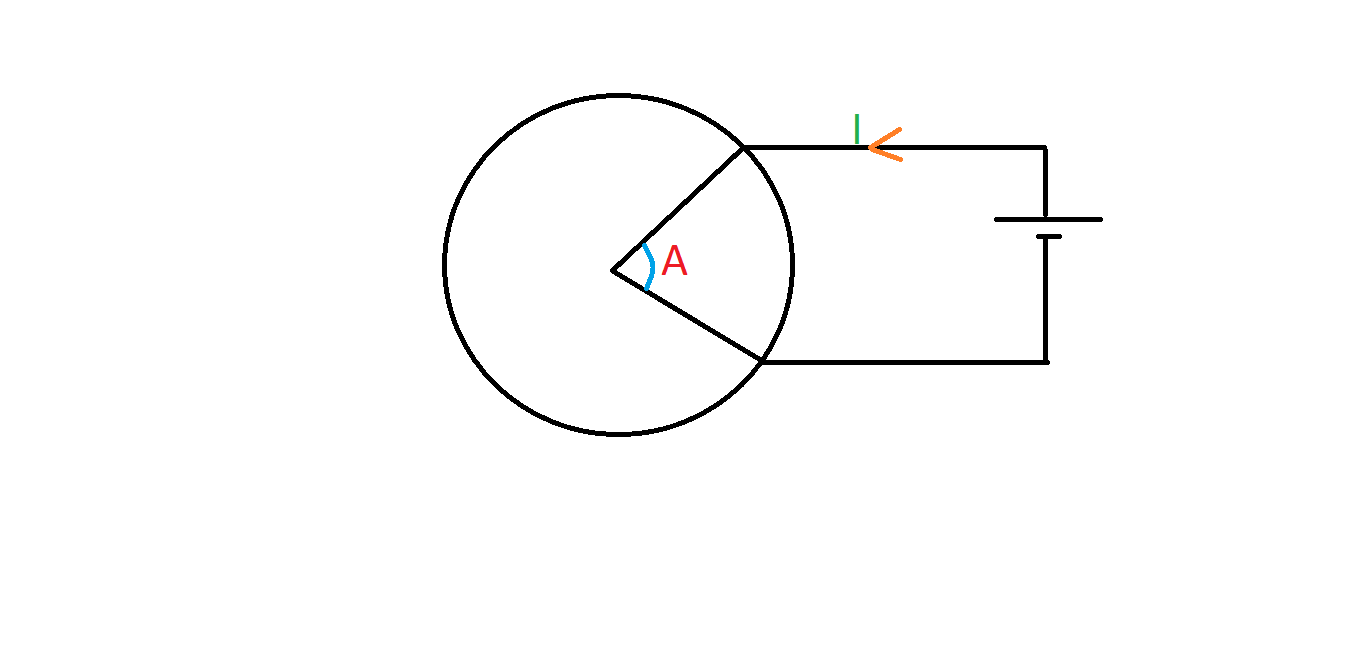# Magnetism in ring

A uniform circular ring conductor is connected to a battery, as shown.

Here the radius of the ring is $5$ meters and the current $I$ is $5$ amperes. If $\angle A = \frac{\pi}{8},$ find the resulting magnetic field intensity at the center of the ring due to the conductor.